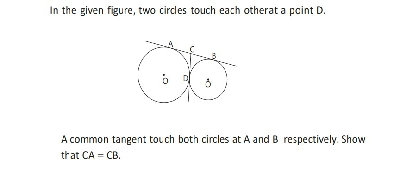"
">

# In the given figure, two circles touch each other at a point $D$.A common tangent touch both circles at $A$ and $B$ respectively. Show that $CA=CB$."

Given: In the given figure, two circles touch each other at a point $D$.A common tangent touch both circles at $A$ and $B$ respectively.

To do: To show that $CA=CB$.

Solution:$\because CA$ and $CD$ are tangents on the points $A$ and $D$ to the circle with centre $O$ from the point $C$.

$\therefore CA=CD\ ......\ ( i)$ [$\because$ tangents drawn to a circle from an external point are equal.]

Similarly, $CB$ and $CD$ are tangents drawn to the circle with centre $O'$ from the point $C$.

Therefore, $CB=CD\ ......\ ( ii)$

From $( i)$ and $( ii)$

$CA=CB=CD$

Thus, it has been proved that $CA=CB$.

Updated on: 10-Oct-2022

23 Views